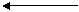Arrays and Functions

What is printed by the following C++ program?

#include <iostream>

#include <cstring>

using namespace std;

int

main()

{

char alphabet = {'a','b','c','d','e','f','g','h','i','j','k',

'l','m','n','o','p','q','r','s','t','u','v','w','x','y','z'};

int i;

for (i = 0; i < 26; i++)

cout << alphabet[i] << ' ';

cout << endl;

for (i = 0; i< 26;i++)

{

alphabet[i] = toupper(alphabet[i]);

cout << alphabet[i]<<' ';

}

cout << endl;

return 0;

}

a b c d e f g h i j k l m n o p q r s t u v w x y z

A B C D E F G H I J K L M N O P Q R S T U V W X Y Z

Press any key to continue

Individual array elements are passed to functions in the same manner as any other variable or value.  They may not, however, be used as formal parameters in a function prototype.

//incorrect

void function (int num);  //not allowed – error

//correct

void function (int num);

void function (int);

Problem:  Write a function plustwo that accepts an integer as its input and returns that value increased by two.  Pass plustwo the elements of a six element array and print the results from main.

#include <iostream>

using namespace std;

void plustwo(int &);

int

main()

{

int numbers, i;

for (i = 0; i < 6; i++)

{

numbers[i] = i+12;

cout << numbers[i] << ' ';

}

cout << endl;

plustwo(numbers);

plustwo(numbers);

for (i = 0; i < 6; i++)

cout << numbers[i] << ' ';

cout << endl;

return 0;

}

void plustwo (int &n)

{

n = n + 2;

}

12 13 14 15 16 17

12 15 14 15 18 17

Press any key to continue

An individual array element can be used in all the same ways simple variables and/or values of the same data type can be used.  An individual array element can be used as an output parameter.  You must remember to include the array subscript.

How can we pass an entire array as an actual parameter?

An array name with no subscript is interpreted by the compiler and operating system as equating to the address of the first element of the array.

 junk4 junk    memory location 14541 junk 5 junk 8 junk 75 junk 45

Note that when passing an array to a function in this manner we are manipulating the original array and NOT a copy.

If we wish to make sure that there are no changes to the array, we precede its definition in the formal parameter list with the keyword const.

Problem:  Write a C++  program that contains a function that will find the smallest element in a six element array.

#include <iostream>

using namespace std;

int find_small (const int num[], int size);

int

main()

{

int numbers1  = {45,23,48,65,72,12};

int numbers2  = {8,32,95,44,-62,56,11,-2};

int small;

small = find_small(numbers1, 6);

cout << "The smallest number in the array is: " << small << endl;

small = find_small(numbers2, 8);

cout << "The smallest number in the array is: " << small << endl;

return 0;

}

int find_small (const int num[], int size)

{

int i;

int tiny;

tiny = num;

for (i = 1; i < size; i++)

if (num[i] < tiny) tiny = num[i];

return tiny;

}

In each function call num receives the address of the first element of the array passed to it.

What is the advantage of passing arrays by reference?  Arrays can be large.  Copying the array can be costly with respect to memory.